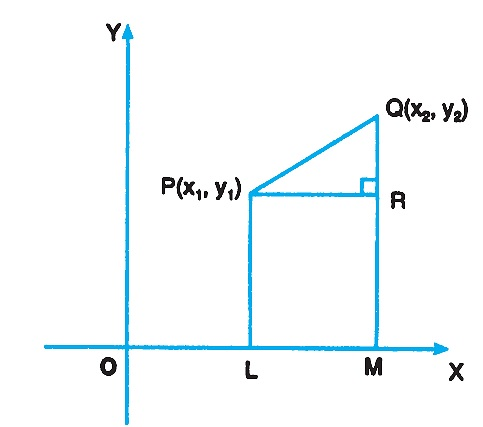# Distance Between Two Points

The distance between any two points P(x1, y1) and Q(x2, y2) in the plane is the length of the line segment PQ.

From P, Q draw PL and QM perpendicular on the x-axis and PR perpendicular on QM. Then,

OL = x1, OM = x2, PL = y1 and QM = y2

PR = LM = OM - OL = x2 - x1

QR = QM - RM = QM - PL = y2 - y1Since PQR is a right angled triangle, by Pythagoras Theorem

$$PQ^2 = PR^2 + QR^2$$

$$PQ^2 = (x_2 - x_1)^2 + (y_2 - y_1)^2$$

$$PQ = \sqrt{(x_2 - x_1)^2 + (y_2 - y_1)^2}$$

$$\text{ Distance between two points} = \sqrt{\text{(difference of abscissae)}^2 + \text{(difference of ordinates)}^2}$$

### Distance of Point from Origin

The distance of the point (x1, y1) from the origin (0, 0) is

$$= \sqrt{x_1^2 + x_2^2}$$